# Almost Periodic Function

(redirected from Almost periodicity)
The following article is from The Great Soviet Encyclopedia (1979). It might be outdated or ideologically biased.

## Almost Periodic Function

a function whose value is approximately repeated when its argument is increased by properly selected constants (the almost periods). More precisely, a continuous function f(x) defined for all real values of 5 x is called almost periodic if for every > 0 there exists an l = l (∊) such that in every interval of length l on the x-axis at least one number τ = τ(∊) can be found for which the inequality ǀf (x + τ) – f(x)ǀ < ∊ is satisfied for all x. The numbers τ are called the almost periods of the function f(x). Periodic functions are special cases of almost periodic functions; simple examples of almost periodic functions that are not periodic can be obtained by adding periodic functions with incommensurable periods—for example, cos x + cos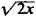.

The following are some important properties of almost periodic functions:

(1) An almost periodic function is bounded and uniformly continuous on the entire x-axis.

(2) The sum and product of a finite number of almost periodic functions is an almost periodic function.

(3) The limit of a uniformly convergent sequence of almost periodic functions is an almost periodic function.

(4) Every almost periodic function has a mean value (over the entire x-axis):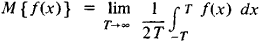(5) To every almost periodic function we can associate a Fourier series: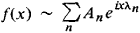where λ1, λ2, …, λn, … can be any sequence of distinct real numbers and

An = M {f (x)enx}

(6) The Parseval equality: for every almost periodic function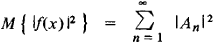(7) Uniqueness theorem: if f(x) is a continuous almost periodic function and if for all real λ

M {f (x)eiλx} = 0

then f(x) ≡ 0. In other words, a Fourier series uniquely determines an almost periodic function.

(8) Approximation theorem: for every ∊ > 0, there exists a finite trigonometric polynomial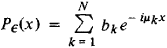(where μκ is a real number) such that the inequality ǀf(x) – P (x)ǀ < ∊ is satisfied for all values of x; conversely, every function f(x) with this property is an almost periodic function.

The first construction of almost periodic functions was given by the Danish mathematician H. Bohr in 1923. Even earlier, in 1893, the Latvian mathematician P. Bohl studied a special case of almost periodic functions—quasi-periodic functions. A new construction of the theory of almost periodic functions was provided by N. N. Bogoliubov in 1930. The theory of almost periodic functions was generalized to include discontinuous functions first by V. V. Stepanov in 1925 and subsequently by H. Weyl and A. S. Besicovitch. A generalization of a different kind was given by the Soviet mathematician B. M. Levitan in 1938.

### REFERENCES

Bohr, H. Pochti periodicheskie funktsii. Moscow-Leningrad, 1934. (Translated from German.)
Levitan, B. M. Pochti-periodicheskie funktsii. Moscow, 1953.
References in periodicals archive ?
The concept of almost automorphy, which is an important generalization of the classical almost periodicity, was first introduced in the literature [1-4] by Bochner in relation to some aspects of differential geometry.
By Theorem 18, some known assertions concerning inheritance of asymptotical periodicity, almost asymptotical almost periodicity and asymptotical almost automorphy under the action of finite convolution products ([5,16]), and the assertions (a)-(b) clarified above, this yields the following result (the uniqueness of solutions follows from the fact that [12, Theorem 2.10.7] holds in degenerate case and that the condition ([diamond]) stated in the formulation of [12, Proposition 2.10.3] holds true, which can be verified by performing the Laplace transform).
On the one hand, due to the fact that almost periodic phenomena exist in the real world, more and more scholars are interested in the almost periodicity and its various generalizations.
Then, from the almost periodicity of [a.sub.ij], [b.sub.ij], [[beta].sub.im], [[gamma].sub.im] and [[tau].sub.im], we can select a sequence {[t.sub.k]} [right arrow] +[infinity] such that
In addition, the almost automorphy, which was introduced in the literature by Bochner in 1955 [7, 8], is a generalization of the almost periodicity and plays an important role in understanding the almost periodicity.
In chapters on stability and boundedness, almost periodicity, and applications, they discuss such matters as the stability of integral manifolds, the Lyapunov method for almost periodic solutions, and fractional impulsive biological models.
Since the solution of system (4) is a piecewise continuous function with points of discontinuity of the first kind [t.sub.k], k [member of] Z, we adopt the following definitions for almost periodicity.
Namely, in order to clarify the problem of the existence of almost periodicity (Bohr), we shall notice that the function f(t), the sum of the series (3.2), has almost periods.
By the almost periodicity of {[a.sub.i](k)}, {[b.sub.i](k)}, and {[c.sub.ij](k)}, there exists an integer valued sequence {[[delta].sub.p]} with [[delta].sub.p] [right arrow] [infinity] as p [right arrow] [infinity] such that
Minimal such function f is regulator of almost periodicity of the sequence A.
present recent methods of study on the asymptotic behavior of solutions of abstract differential equations in Banach spaces, such as stability, exponential dichotomy, periodicity, almost periodicity, and almost automorphy of solutions.
The concept of pseudo almost periodicity is a natural genelization of almost periodicity.

Site: Follow: Share:
Open / Close